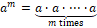## Weekly Overview

Weekly Topics

The focus of this week’s instruction is to deepen students’ understanding of:

• Interpreting Division of a Fraction by a Whole Model and Visual Models
• Interpreting and Computing Division of a Fraction by a Fraction and Visual Models.
• Exponents
• The Order of Operations
• Replacing Letters with Numbers

Materials Needed

• Counters
• Fraction Tiles (see example of usage in Representations below)
• Tape Diagrams
• Basic Calculator

Standard(s) Covered

6.NS.A.1 Interpret and compute quotients of fractions, and solve contextual problems involving division of fractions by fractions (e.g., using visual fraction models and equations to represent the problem is suggested).

6.EE.A.2 Write, read, and evaluate expressions in which variables stand for numbers.

1. Write expressions that record operations with numbers and with variables. For example, express the calculation "Subtract y from 5" as 5 - y.
2. Evaluate expressions at specific values of their variables. Include expressions that arise from formulas used in real-world problems. Perform arithmetic operations, including those involving whole number exponents, in the conventional order when there are no parentheses to specify a particular order (Order of Operations).

RepresentationsAdditional Terms and Symbols

• Multiplicative Inverses (A multiplicative inverse of a number is a number such that the product of both numbers is 1.  For example, $${3 \over 4}$$ and $${4 \over 3}$$ are multiplicative inverses of one another because $${3 \over 4}$$ x $${4 \over 3}$$ = $${4\over 3}$$ x $${3 \over 4}$$ = 1. Multiplicative inverses do not always have to be the reciprocal.  For example, $${1\over 5}$$ and $${10\over 2}$$ both have a product of 1, which makes them multiplicative inverses of each other.)
• Exponential Notation for Whole Number Exponents (Let m be a nonzero whole number.  For any number a, the expression am is the product of m factors of a (i.e.,  =).  The number a is called the base, and m is called the exponent or power of a.)
• Variable (A variable is a symbol (such as a letter) that is a placeholder for a number.)

Familiar Terms

• Expand
• Properties of Operations (distributive, commutative, associative)
• Variable or Unknown Number